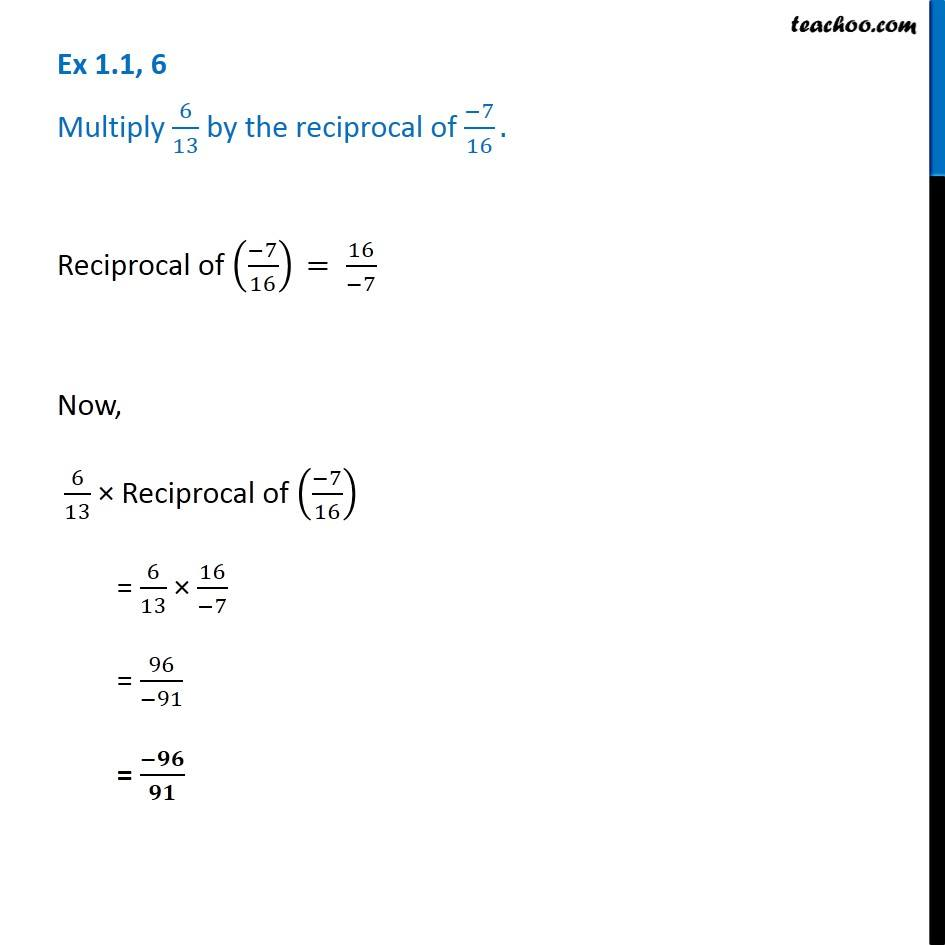Ex 1.1

Chapter 1 Class 8 Rational Numbers
Serial order wiseGet live Maths 1-on-1 Classs - Class 6 to 12

### Transcript

Ex 1.1, 6 - Chapter 1 Class 8 NCERT Book - Rational Numbers Multiply 6/13 by the reciprocal of (−7)/16. Reciprocal of ((−7)/16) = 16/-7 Now, 6/13 × Reciprocal of ((−7)/16) = 6/13 × 16/(−7) = 96/(−91) = -96/91